# 12-267/Numerical Methods

## Summary of Numerical Methods

Based largely off of a note available here posted by Simon1 --Twine 20:55, 25 October 2012 (EDT)

Numerical methods: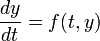$\frac{dy}{dt} = f(t, y)$ and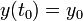$y(t_0) = y_0$,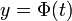$y = \Phi(t)$ is a solution.

1. Using the proof of Picard's Theorem: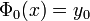$\Phi_0(x) = y_0$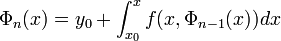$\Phi_n(x) = y_0 + \int_{x_0}^x f(x, \Phi_{n-1}(x)) dx$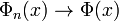$\Phi_n(x) \rightarrow \Phi(x)$

2. The Euler Method: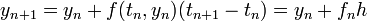$y_{n+1} = y_n + f(t_n, y_n)(t_{n+1} - t_n) = y_n + f_n h$ if h is constant

Backward Euler formula: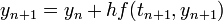$y_{n+1} = y_n + h f(t_{n+1}, y_{n+1})$

Local truncation error: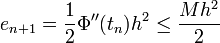$e_{n+1} = \frac{1}{2} \Phi''(t_n)h^2 \leq \frac{Mh^2}{2}$ where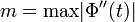$m = \mathrm{max} |\Phi''(t)|$

Local error is proportional to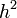$h^2$.

Global error is proportional to h.

3. Improved Euler Formula (or Heun Formula):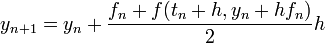$y_{n+1} = y_n + \frac{f_n + f(t_n + h, y_n + hf_n)}{2} h$

To determine local error we took the taylor expansions of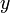$y$ and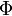$\Phi$ and compared them.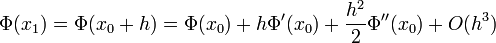$\Phi(x_1) = \Phi(x_0 + h) = \Phi(x_0) + h \Phi'(x_0) + \frac{h^2}{2} \Phi''(x_0) + O(h^3)$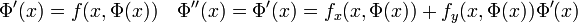$\Phi'(x) = f(x, \Phi(x)) \quad \Phi''(x) = \Phi'(x) = f_x(x, \Phi(x)) + f_y(x, \Phi(x))\Phi'(x)$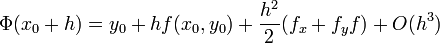$\Phi(x_0 + h) = y_0 + h f(x_0, y_0) + \frac{h^2}{2}(f_x + f_y f) + O(h^3)$

We compare this to the computed value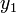$y_1$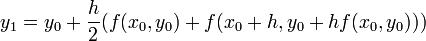$y_1 = y_0 +\frac{h}{2}(f(x_0, y_0) + f(x_0 + h, y_0 + hf(x_0, y_0)))$

Taking the taylor expansion we get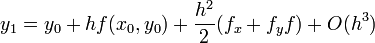$y_1 = y_0 + h f(x_0, y_0) + \frac{h^2}{2}(f_x + f_y f) + O(h^3)$

So we have shown that the Improved Euler Formula is accurate up to an error term proportional to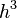$h^3$

Local truncation error is proportional to$h^3$

Global truncation error is proportional to$h^2$

4. The Runge-Kutta Method: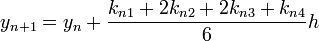$y_{n+1} = y_n + \frac{k_{n1} + 2k_{n2} + 2k_{n3} + k_{n4}}{6} h$

where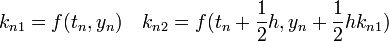$k_{n1} = f(t_n, y_n) \quad k_{n2} = f(t_n + \frac{1}{2} h, y_n + \frac{1}{2}hk_{n1})$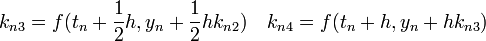$k_{n3} = f(t_n + \frac{1}{2} h, y_n + \frac{1}{2}hk_{n2}) \quad k_{n4} = f(t_n + h, y_n + hk_{n3})$

Local truncation error is proportional to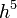$h^5$.

Global truncation error is proportional to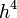$h^4$.

## Python Example of Euler's Method

In class on October 15th we discussed Euler's Method to numerically compute a solution to a differential equation.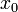$x_0$ and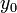$y_0$ are given as well as an increment amount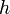$h$,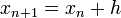$x_{n+1} = x_n + h$, and we use the guess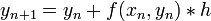$y_{n+1} = y_n + f(x_n, y_n)*h$ where f computes the derivative as a function of x and y.

Here is an example of code (written in Python) which carries out Euler's Method for the example we discussed in class,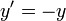$y' = -y$:

   def f(x, y):
return -y

def euler(x, y, f, h, x_max):
"""Take in coordinates x and y, a function f(x, y) which calculates
dy/dx at (x, y), an increment h, and a maximum value of x.

Return a list containing coordinates in the Euler's Method computation
of the solution to Phi' = f(x, Phi(x)), Phi(x) = y, with the x
values of those coordinates separated by h, and not exceeding x_max.
"""
if x > x_max: # we have already calculated all our values
return []
x_next, y_next = (x + h, y + f(x, y)*h) # calculate the next x, y values
# return the current coordinates, and every coordinates following it, in a list
return [(x_next, y_next)] + euler(x_next, y_next, f, h, x_max)

if __name__ == '__main__':
print euler(0, 1, f, 0.01, 1)[-1]